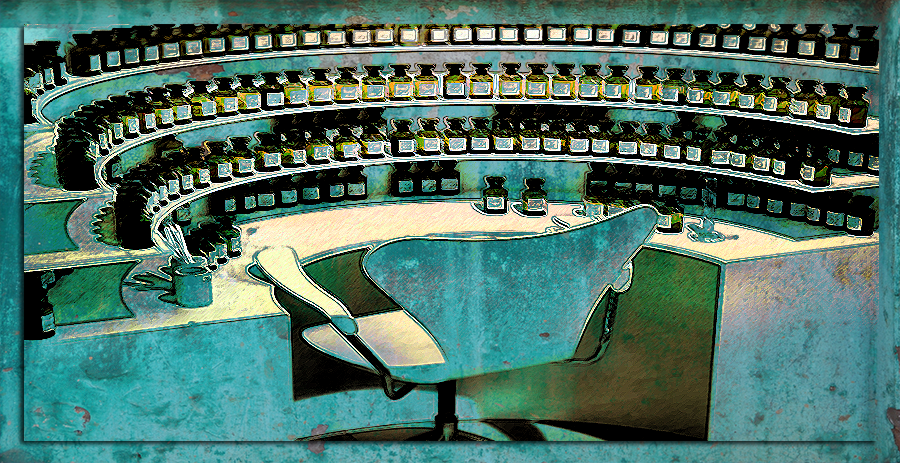# Calculations## CALCULATIONS

Cologne = 3 to 10%   EDT = 10 to 15%   EDP = 15 to 20%   Parfum = 20% +

Before blending your oils, weigh the empty beaker you will be mixing your formula in. Make a note of the weight. Once you reach completion and you are happy with your creation you must weigh the beaker again containing the perfume formula. Now, deduct the weight of the empty beaker from the weight of the occupied one. The answer gives you the weight of your perfume formula.

In order to find out how much alcohol you must add, you first decide what strength you desire your perfume to be. For instance, if you want to produce an Eau de Parfum, you require your final perfume to be 15 to 20% oils and 80 to 85% Alcohol.

To work this out you take the weight of the perfume formula and divide it by varying numbers (which represent the weight of an overall volume) until you come up with an answer ranging from 15 to 20%. For instance, say your perfume formula weighs 7g, and you divide it by 30 (30ml) your answer would be 0.23, which would place your perfume at a 23% concentration. This is slightly over the percentage of an Eau de Parfum, but if you are happy with that number, you would simply subtract 7 from 30, giving you 23. That means for a 23% strength perfume you need to add 23g of alcohol to your 7g of perfume oils to give a total weight of 30g or a volume of 30ml.

7(g) ÷ 30(ml) = 0.23%          30(ml) – 7(g) = 23(g)          23(g) + 7(g) = 30(ml) @ 23%

If you are working in drops and you decide that you would like to make 30ml of a finished perfume you would take into account that 1ml is approximately 20 drops. 30 x 20 = 600 (drops). Next, you would need to decide on the strength of the perfume, say 20% for example and calculate what 20% of 600 is. The answer is 120 (drops). Therefore you would need to add 120 drops of perfume oils to 480 drops of alcohol to get a 30ml bottle of perfume at a strength of 20%.

Bear in mind that working in drops is not accurate, and results can differ with each batch, as oils have various levels of viscidity which, means that the weight of drops will vary. In my experience, you get inconsistent results.

I created a perfume concentrate using the drops method for a 30ml bottle of finished perfume. That means I used 120 drops of oil, but instead of adding 480 drops of alcohol for a supposed 20% strength perfume, I divided the weight of the perfume concentrate (1.656g) by the volume of my perfume bottle (30ml) which, gave me the strength of 5%, not 20%.

In the end, I settled at a volume of 10ml which gave me a finished perfume at a strength of 16%. So as you can see, making perfume by drops is not a good practice.